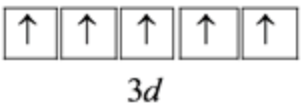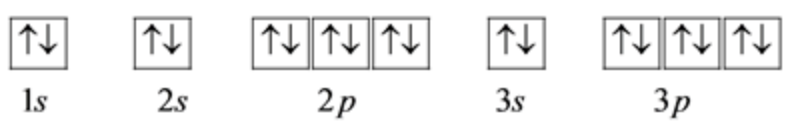In: Chemistry

In the ground-state electron configuration of Fe3+, how many unpaired electrons are present?

Part A

In the ground-state electron configuration of Fe3+, how many unpaired electrons are present?

Part B

Build the orbital diagram for the ion most likely formed by phosphorous.
Use the buttons at the top of the tool to add orbital's in order of increasing energy, starting at the bottom with the lowest energy orbitals. Click within an orbital to add electrons.

Solutions

Expert Solution

Concepts and reason

The electrons are filled in the orbitals according to Hund's rule. It states that the pairing of electrons takes place only after all the degenerate orbitals are half-filled. If an atom loses electrons, it gets a positive charge, and if the atom gains electrons, it gets a negative charge.

Fundamentals

The ground state is the molecule's energy state when all the electrons are present in the lowest possible molecular orbitals. In an excited state, one electron goes from lower energy orbital to higher energy molecular orbital.

$$\mathbf{A}$$

The atomic number of Fe is 26.

It loses 3 electrons to form $$\mathrm{Fe}^{3+}$$ ion.

Hence, the number of electrons in $$\mathrm{Fe}^{3+}$$ is 23 . Therefore, its ground state electron configuration is,

$$1 s^{2} 2 s^{2} 2 p^{6} 3 s^{2} 3 p^{6} 3 d^{5}$$

Write the orbital configuration for the outermost $$3 \mathrm{~d}$$ orbital as follows:Hence, the number of unpaired electrons in $$\mathrm{Fe}^{3+}$$ are 5 .

In the $$3 \mathrm{~d}$$ subshell, there are 5 degenerate $$\mathrm{d}$$ orbitals. Hence, according to Hund's rule, each orbital gets one electron and a total of 5 unpaired electrons.

$$\mathbf{B}$$

The most likely ion formed by phosphorous is $$\mathrm{P}^{3-}$$.

The electron configuration of $$\mathrm{P}^{3-}$$ is,

$$1 s^{2} 2 s^{2} 2 p^{6} 3 s^{2} 3 p^{6}$$

The orbital diagram for $$\mathrm{P}^{3-}$$ is as follows:The atomic number of phosphorous is 15. Its electron configuration is,

$$1 s^{2} 2 s^{2} 2 p^{6} 3 s^{2} 3 p^{3}$$

It needs 3 electrons to attain an inert gas configuration.

Hence, the most likely ion formed by phosphorous is $$\mathrm{P}^{3-}$$.

Related Solutions

How many electrons did it lose in the process of acquiring this charge?
When a honeybee flies through the air it develops a charge of +20pC. How many electrons did it lose in the process of acquiring this charge? Express answer as a number
how many electrons in an atom could have these sets of quantum numbers?
How many electrons in an atom could have these sets of quantum numbers? n=2 --> number electrons = ? n=5, l=2 --> number electrons= ? n=7, l=1, ml=-1 --> number electrons= ?
How many electrons in an atom could have these sets of quantum numbers? n=3
How many electrons in an atom could have these sets of quantum numbers? (a) n=3 (b) n=4, l=2 (c) n=7, l=3, ml=-1
At the end of the electron transport chain the electrons are taken up by oxygen to generate
At the end of the electron transport chain the electrons are taken up by oxygen to generate  ATP heat glucose water
How many Subshells are in the N shell?
How many subshells are in the n = 3 shell? How many orbitals are in the n = 3 shell?  What Is the maximum number of electrons in the n = 3 shell?
Classify each orbital diagram for ground-state electron configurations by the rule or principle it violates.
Part A Classify each orbital diagram for ground-state electron configurations by the rule or principle it violates. Drag the appropriate items to their respective bins.
How many counties are there in our country Kenya?
How many counties are there in our country Kenya?
How many counties are there in our country Kenya?
How many counties are there in our country Kenya?
How many counties are there in our country Kenya?
How many counties are there in our country Kenya?
How many moles of zinc are in 4.80 kg of zinc?
± Introduction to Electroplating Learning Goal: To relate current, time, charge, and mass for electroplating calculations. Electroplating is a form of electrolysis in which a metal is deposited on the surface of another metal. To quantify electrolysis, use the following relationships. Electric current is measured in amperes (A), which expresses the amount of charge, in coulombs (C), that flows per second (s): 1 A=1 C/s Another unit of charge is the faraday (F), which is equal to a mole of...